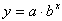Writing a Function

An exponential function is written in the form. Recall from the last lesson that the b-value is the common ratio and the a-value is the initial value. On a graph, the a-value will represent the y-intercept since any number raised to the power of zero equals one. If either the y-intercept or the common ratio are known on the graph, you can write a function.

Listen